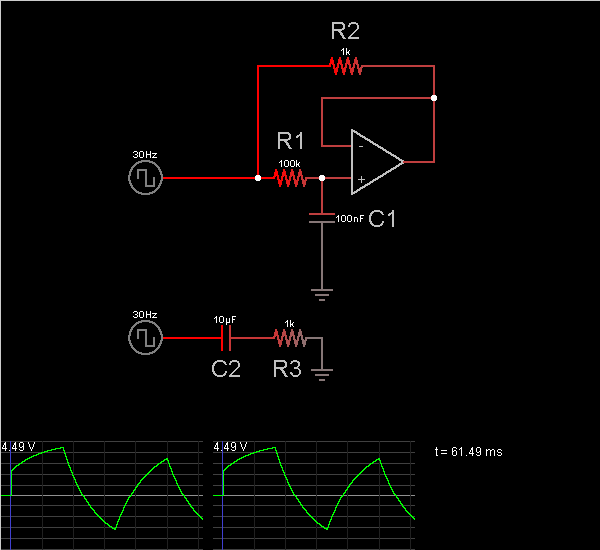# Circuit Simulator - Capacitance Multiplier

## Where can I get Capacitance Multiplier Circuit Diagram with Explanation?

IndiaBIX provides you lots of fully solved Capacitance Multiplier circuit diagram with detailed explanation and working principles.

## How to design a Capacitance Multiplier (electronic circuit)?

You can easily design the Capacitance Multiplier circuit by practicing the exercises given below. Here you can design and simulate your own electronic circuits with this Online Circuit Designer and Simulator.### Circuit Description:

The circuit on top uses an op-amp and a small capacitor to simulate a much larger capacitor. It simulates the circuit on the bottom; the resistor R2 is the same size as the resistor in the circuit being simulated (R3), but the capacitor C1 is 100 times smaller than C2.

Current flows from the input source through R1 to the capacitor (C1). Since R1 is 100 times larger than R2, there is 1/100th the current through it into the capacitor. For a given input voltage, the rate of change in voltage in C1 is the same as in C2, because C2 has 100 times the capacitance to make up for 1/100th the current.

So the voltages across the two capacitors are the same, but the currents are not. The op-amp causes the – input to be held at the same voltage as the voltage across C1. This means R2 has the same voltage across it as R3, and therefore the same current. -- Credits: Mr. Paul Falstad.

 Mike said: (Sep 10, 2014) Hi. I was just wondering, Does the other terminal of the signal source connect to ground, And, does the op amp need a load resistor between the output and ground to provide a current return path back to the signal source, I can't see how the higher current running through the feedback circuit gets back to signal source. Thanks.